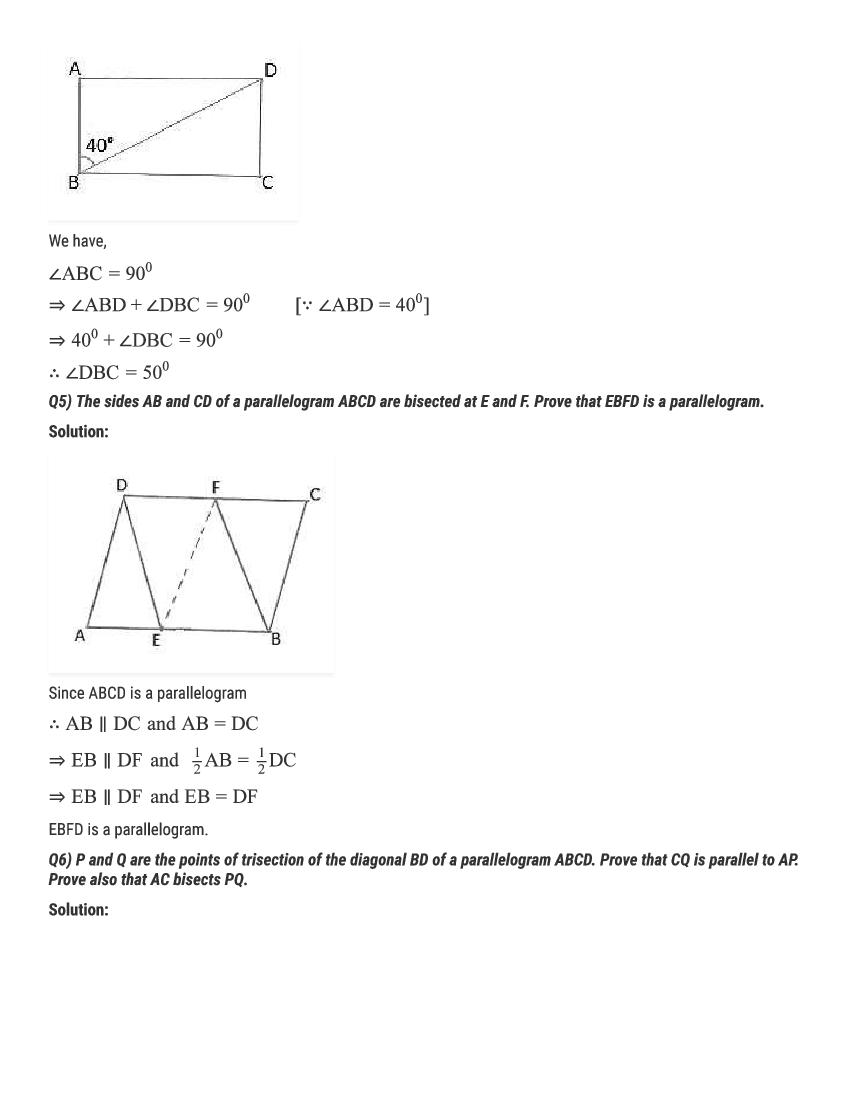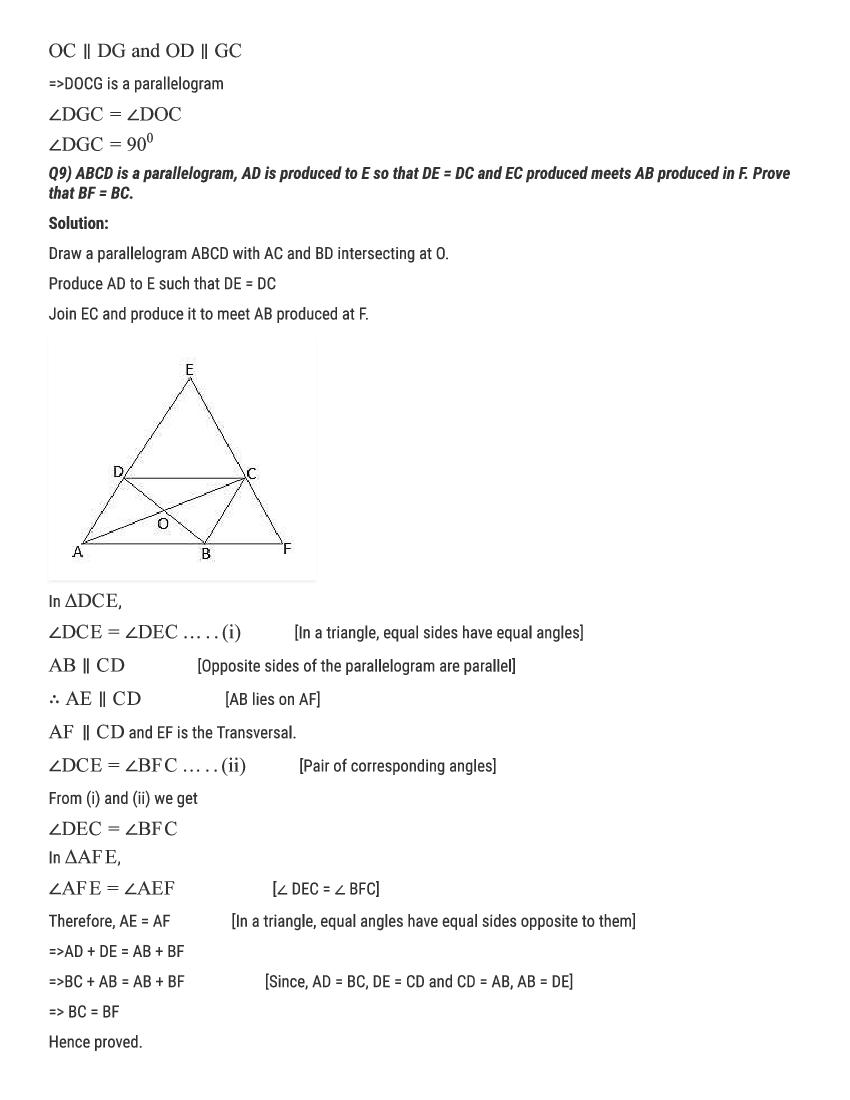# RD Sharma Solutions Class 9 Chapter 14 Quadrilaterals Exercise 14.3

Here you can get free RD Sharma Solutions for Class 9 Maths Chapter 14 Quadrilaterals Exercise 14.3. All RD Sharma Book Solutions are given here exercise wise for the chapter Quadrilaterals. RD Sharma Solutions are helpful in the preparation of several school level, graduate and undergraduate level competitive exams. Practicing questions from RD Sharma Mathematics Solutions for Class 9 Chapter 14 Quadrilaterals is proven to enhance your math skills.

 Class: Class 9th Chapter: Chapter 14 Exercise: Exercise 14.3 Name: Quadrilaterals

## RD Sharma Solutions Class 9 Chapter 14 Quadrilaterals Exercise 14.3

RD Sharma Class 9 Solutions Chapter 14 for Quadrilaterals Exercise 14.3 are given below.

RD Sharma Solutions Class 9 Chapter 14 Quadrilaterals Excercise 14.3